Categories
Pages
-## Bouncing robots and deformed planets

November 22nd, 2014 | by

On a long hiking trip we were bored on our way back. So what do you do if you are a physicist and if you are outside and have no data and nothing to look up? You bring up some Fermi-Problem to solve as a pastime. Our up-to-date problem chosen was concerning the marvelous landing of Philae on the comet 67P/Churyumov–Gerasimenko. After the first “landing” the little robot bounced back and it took it around 2 hours to touch the comet again (resulting in at least one more bounce). The question at hand is: How far up did the robot bounce? We tried to answer this question, but this lead us to some more questions with quite unintuitive results.

For solving the Fermi-Problem, we need some basic input, so we tried to remember some facts about the comet. The head of the rubber-duck-shaped comet is around 2 km in diameter, and the larger part is around 5 km in diameter. The average density is 0.4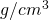, compared to the earth’s density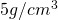. So we can make as a first assumption that the comet is a sphere (hm?) in vacuum (check) with a diameter of about 3 km (averaged from the head and the body). We can estimate the gravitational acceleration of the comet from that of the earth by taking the ratio of the radii and the densities: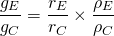If you solve this equation for the acceleration of the comet and insert the numbers you get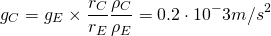Since we were on a hiking trip, we had to assume that the acceleration remains constant while moving away from the comet. This is a rough assumption, but let’s see where it gets us. The height is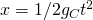andis the time, the robot fell, so roughly an hour, 3600 seconds. So the height is about 1.3 km. When I got home, I looked the actual height up, which was roughly 1 km. Comparing those numbers satisfied me, since with the constant acceleration I used, the estimated height should be higher then the actual height.

But then I found something confusing: I looked up the actual value for the acceleration on the surface of the comet and I found some references giving a number of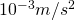which is a factor of 5 larger then the one I got from my estimation (spherical comet in vacuum). This is weird, the acceleration we got from comparing with the earth was a factor of 5 smaller then the actual one? What is confusing here is not the factor 5, but that the estimation with the sphere gives a smaller acceleration then the rubber-duck-shape? Can the gravitational force increase  if you have a sphere with a specific density and a mass and you deform it into another shape?

So the best thing to check this was to calculate the gravitational force of an ellipsoid to see, how the gravitation changes if you go away from the sphere-shape. Since it is simpler to calculate, I put the test mass on the top of it , so that this reference point is invariant under rotation along this axis. Then there are two cases: The oblate ellipsoid, which has the form of a M&M and the test mass sits on the flat side of the M&M (I marked the position of the test mass with a green dot in the figure below), and the stretched form which resembles a cigar, where the test mass sits on the pointy side of the cigar. After calculating the gravitational potential and the force, we are left with this plot: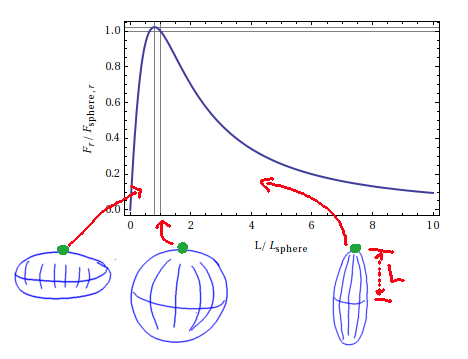On the x-axis you see here the length of the shape that is stretched (distance from the equator to the pole). For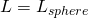, you get the sphere. On the y-axis you see the radial force (normalized to the radial force on a sphere). It is surprising that the forces is not maximal for the sphere, but for a oblate spheroid close to the sphere. The ratio of forces I get for the maximal value is around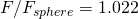. This is pretty close the result for an arbitrary (but symmetric) deformation of  a body, see e.g. here and here, which have. So the gravitational force can actually become larger if you deform a sphere into a oblate ellipsoid! This was quite surprising to me. Some further calculation showed in contrast, that you cannot increase the gravity by sitting on the side of the body (so along the equator), and that the sphere will always give you the largest gravity (for fixed mass and density). This results also in the fact that the gravity on the (oblate) earth is larger on the poles then on the equator.

This was in interesting result, however, it does not explain the large factor between Tschuris acceleration and the one that can be estimated from a sphere the same size. But as it turns out, the initial value that confused me () was an old value (from 2004, probably where the comet was not known very well), and is cited in many Wiki articles. In the german Wiki-page of Tschuri, a newer value is stated

Die Gravitationsbeschleunigung auf der Oberfläche des Kometen beträgt ca. 1/100.000 der Erdbeschleunigung (0,1 mm/s²). Philae hat 100 kg Masse mit entsprechend großer Trägheit, auf den Lander wirkt jedoch nur eine Gewichtskraft, die der von 1 Gramm auf der Erde entspricht.

which also fits to the estimation, refering to a new video ESA made. I haven’t watched it myself yet, but it will be interesting what they say about the acceleration. However, this new number is a factor 2 smaller then what you get with the estimation above, and that is reasonable.